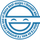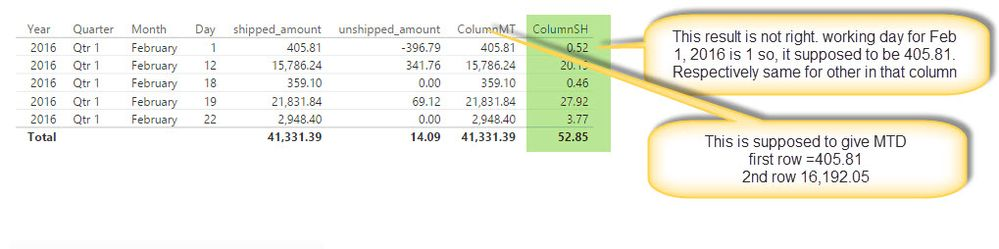cancel
Showing results for
Did you mean:Helper I

## working day, total working day, running total

Below is the link to powerbi file. i need a big help from you..geniuses

Need following columns or measures

ColumnMT= MTD running shipped_amount
Working day (for example for Feb 1 2016, working day is 1, for Feb 8, 2016 it is 6 )
ColumnSH = month to date running shipped amount / working day
ColumnRR = ColumnAAC * Total no of working Days for that selected Year and Month

My Line Chart Axis=working Days, values are columnSH, ColumnRR

How can i achieve this? i have been strugling for a week. When i try to create columns, some function doesn't work. When i try to create measure, i am not able to use some calculated columns.

1 ACCEPTED SOLUTIONSolution Sage

Here. Check the updated file with added measures etc.

I'm still not certain what you want to show in the chart.

I'd suggest you go through each line carefully and verify that calculated values are what you wanted. If not, you'll want to give at least few samples so that either I or anyone else helping you can verify their solution.

16 REPLIES 16Solution Sage

I'd recommend you add date dimention table.

```DimDate =
CALENDAR (
DATE ( YEAR ( MINX ( Table1, Table1[transaction_date] ) ), 1, 1 ),
DATE ( YEAR ( MAXX ( Table1, Table1[transaction_date] ) ), 12, 31 )
)```

`Month = MONTH([Date])`
`Workday = IF(WEEKDAY([Date],2)>5,0,1)`

Then add following measures to Table1:

```ColumnMT =
CALCULATE (
SUM ( Table1[shipped_amount] ),
FILTER (
ALLSELECTED ( DimDate[Date].[Day] ),
DimDate[Date].[Day] <= MAX ( DimDate[Date].[Day] )
)
)```
```WorkingDays =
CALCULATE (
SUM ( DimDate[Workday] ),
FILTER (
ALLSELECTED ( DimDate[Date].[Day] ),
DimDate[Date].[Day] <= MAX ( DimDate[Date].[Day] )
)
)```
`ColumnSH = [ColumnMT]/[WorkingDays]`

However, I'm not sure what you mean by rest of your questions. I was not able to find ColumnAAC in your sample and can't understand how you want to present the data (if you need working days as axis, you'd need it not as measure but as calculated column).Helper IHelper I

You are really a genius 🙂
Sorry, That ColumnACC is ColumnSH

Now for ColumnRR i need to multiply ColumnSH to total no of working days for that month.

Yes i need working days as axis. how do i create calculated column of working days?

🙂Solution Sage

I'm headed out for the night. I'll see if I can find the time tomorrow.Helper I

Thank you!Solution Sage

Not sure if this is what you wanted. I'm having trouble understanding purpose of Column RR.

At any rate, check the file in link.Helper I

WOW..you are very close. One last thing is working days. Working days is considered as Monday to Friday

Today is June 16, 2017, Day =16 but Working Day = 12.  And I am looking working days by month. Is it too much?

Other than that, the solution looks great.Solution Sage

Hmm? Working days should adjust to each month. Remove filter from the visiual and you should see it.

If working days should be calculated regardless of filter. Instead of using the measure, use the calculated column added to DimDate table [RWkday].Helper I

This is what i am getting. Am i doing something wrong?

`TestColumn = [ColumnMT]/RELATED(DimDate[RWkday])`Solution Sage

You shouldn't devide measure by column. You should create another measure to devide it by.

I'm bit busy right now with work. I'll try to look at later it if you haven't solved it by then.Helper I

Sure..i will wait for you. I have no idea how to make it happen. 😞Solution Sage

Here. Check the updated file with added measures etc.

I'm still not certain what you want to show in the chart.

I'd suggest you go through each line carefully and verify that calculated values are what you wanted. If not, you'll want to give at least few samples so that either I or anyone else helping you can verify their solution.Helper I

One thing i noticed that the numbers in the chart do not match.  It works when you put date (day) in the Axis.Solution Sage

That's because you wanted to chart based on Working days.

Since it's just integer and each month there could be duplicate...

I suggest you use actual date column for axis.

Edit: Or alternately create another column, to plot... Ex. Month + Working days.Helper I

Month+Working Days is not going to create duplicate values? How does that work?Helper I

Perfect!! thank you so much.